# RD Sharma Solutions For Class 12 Maths Exercise 19.7 Chapter 19 Indefinite Integrals

RD Sharma Solutions for Class 12 Maths Exercise 19.7 Chapter 19 Indefinite Integrals is provided here. The RD Sharma solutions which contain a huge number of solved examples and illustrations provide stepwise explanations of various difficult concepts and offer a wide variety of questions for practice. The pdf of RD Sharma Class 12 Solutions Maths Chapter 19 Indefinite Integrals Exercise 19.7 is provided here.

Practising these questions will ensure that they can easily excel in their final exams. Students can refer and download this exercise pdf from the below-given links. Here, students will learn to examine the integrals of the form

$$\begin{array}{l}\int \sin mx \cos n x \ dx, \int \sin mx \sin n x \ dx , \int \cos mx \cos n x \ dx\end{array}$$
. To evaluate these types of integrals, we use trigonometrical identities to express products into sums.

## Download the PDF of RD Sharma Solutions For Class 12 Chapter 19 – Indefinite Integrals Exercise 19.7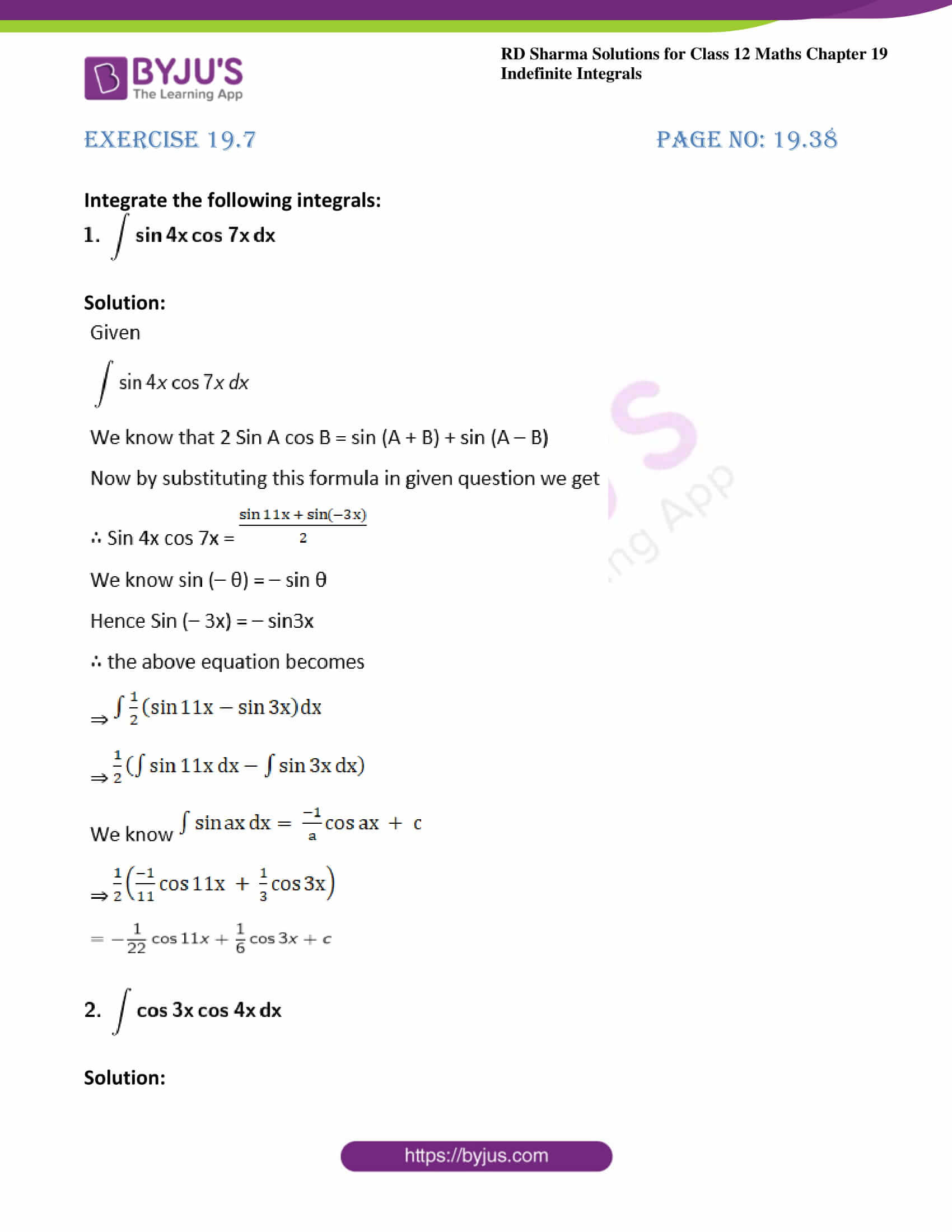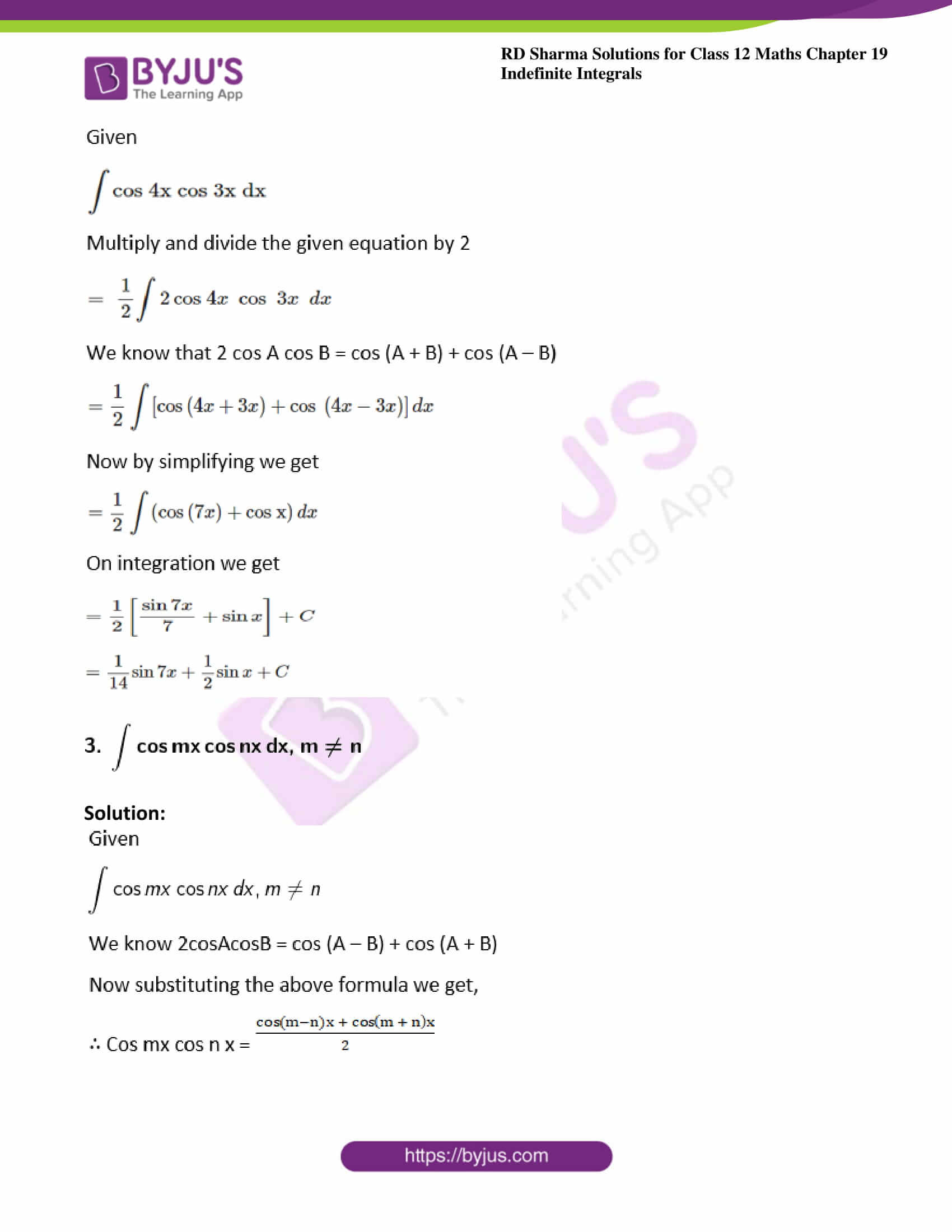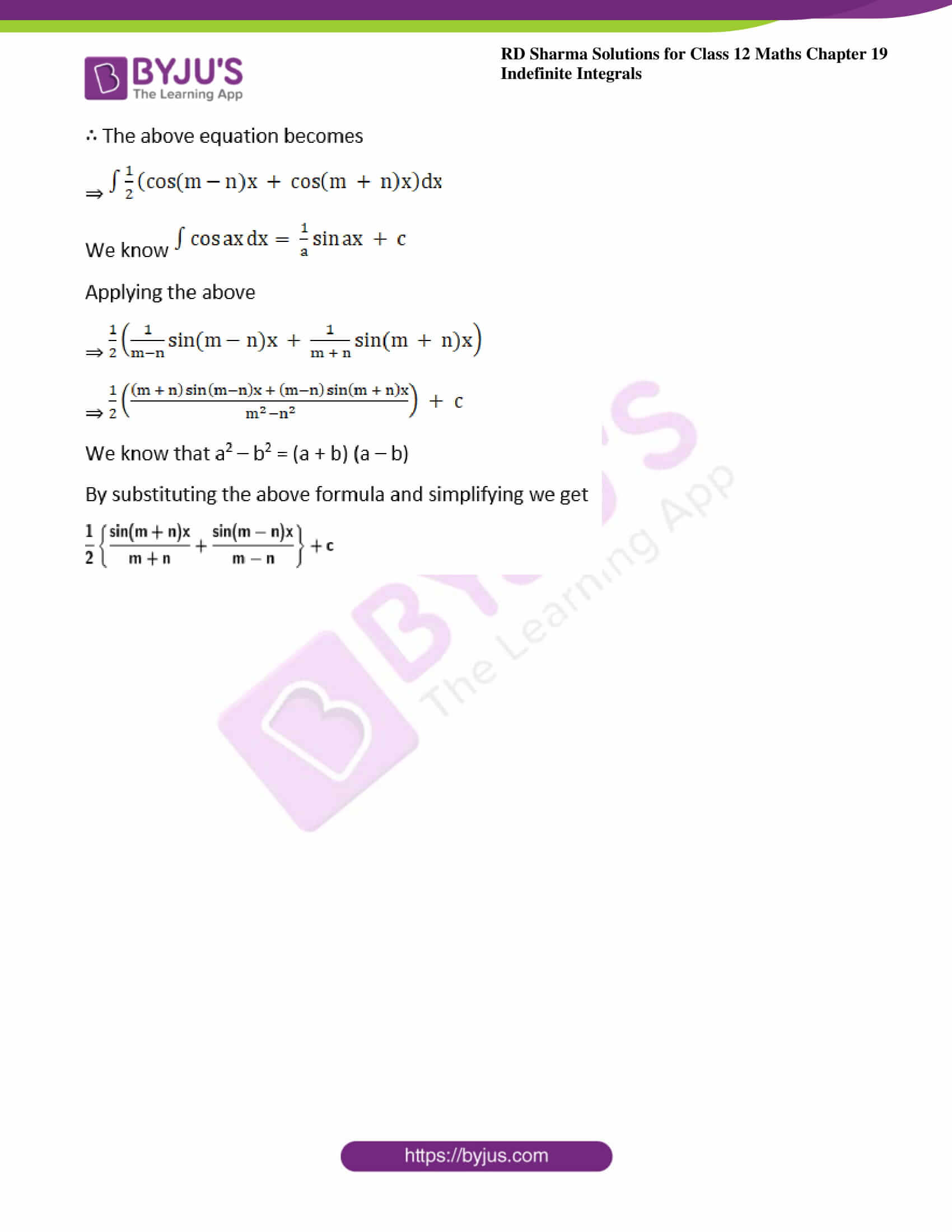### Exercise 19.7 Page No: 19.38

Integrate the following integrals: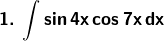Solution: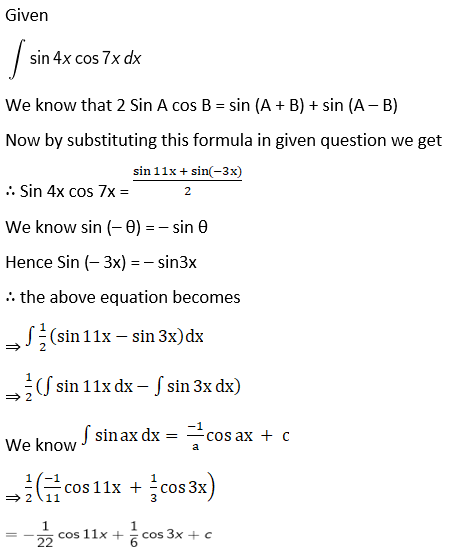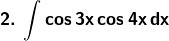Solution: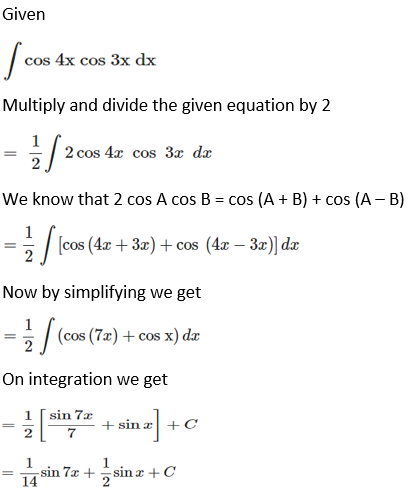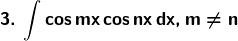Solution: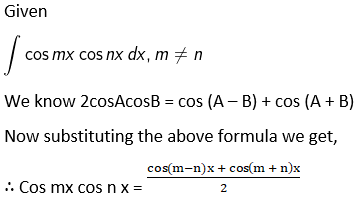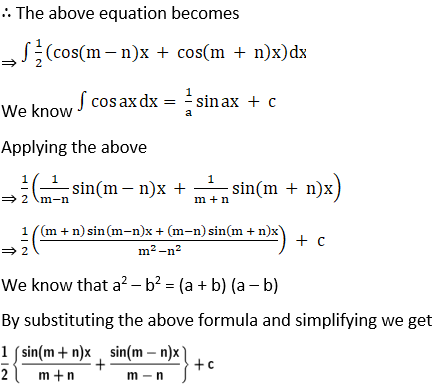### Access other exercises of RD Sharma Solutions For Class 12 Chapter 19 – Indefinite Integrals

Exercise 19.1 Solutions

Exercise 19.2 Solutions

Exercise 19.3 Solutions

Exercise 19.4 Solutions

Exercise 19.5 Solutions

Exercise 19.6 Solutions

Exercise 19.8 Solutions

Exercise 19.9 Solutions

Exercise 19.10 Solutions

Exercise 19.11 Solutions

Exercise 19.12 Solutions

Exercise 19.13 Solutions

Exercise 19.14 Solutions

Exercise 19.15 Solutions

Exercise 19.16 Solutions

Exercise 19.17 Solutions

Exercise 19.18 Solutions

Exercise 19.19 Solutions

Exercise 19.20 Solutions

Exercise 19.21 Solutions

Exercise 19.22 Solutions

Exercise 19.23 Solutions

Exercise 19.24 Solutions

Exercise 19.25 Solutions

Exercise 19.26 Solutions

Exercise 19.27 Solutions

Exercise 19.28 Solutions

Exercise 19.29 Solutions

Exercise 19.30 Solutions

Exercise 19.31 Solutions

Exercise 19.32 Solutions